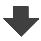Search:

# Examples of electrolysis

## Exercise 2

Electrolysis of a copper(II) sulfate solution (sulfuric acid added) is done between copper electrodes. Give the formulas of the ions which are in presence !

Ions : $Cu^{2+}$ ; $SO_4^{2-}$ from the dissolution and dissociation of copper(II) sulfate; $H^{+}$ from sulfuric acid.

Look for the strongest oxidant - for the strongest reductant !

$Cu^{2+}$ is the strongest oxidant $Cu$ is the strongest reductant.

Write the equations of the reactions occuring at the electrodes !

Cathode: $Cu^{2+}+2e^-\longrightarrow Cu$ (reduction) Anode: $Cu-2e^-\longrightarrow Cu^{2+}$ (oxydation)

What is the use of this reaction ?

The reaction does the electro-refining of copper:1)The less noble metals of the impure anode (stronger reductants) than copper are oxidised to cations and dissolve, but they can't be deposited at the catode. 2)The copper of the impure anode is oxidized to copper(II) ion and dissolves, it is deposited at the cathode. 3)The more noble metals of the anode are not oxidised, they settle out as a deposit of mud to the bottom of the vessel.